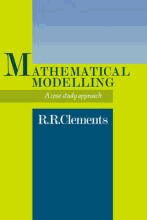# Mathematical Modelling : A Case Study Approach by Clements, Dick

• R 500.00
Unit price per
• Save R 318
Tax included.

ISBN: 9780521089555

CAMBRIDGE UNIVERSITY PRESS | 06 November 2008

Paperback | 176 pages Description

This book, based on a successful undergraduate course designed by the author, describes mathematical modelling and its application to the solution of problems in industry and commerce. In the early chapters of the book the author first sets out a clear introduction to the principles of mathematical modelling, including sections on the various methodologies of mathematical modelling and their relationship to systems methodologies. He then offers some insights into practical aspects of mathematical modelling and proceeds to describe the development and operation of the undergraduate course upon which the book is based. In the later chapters seven case studies, based on real problems contributed by mathematicians working in industry, are introduced. Each chapter describes a problem, the solution originally adopted and variations on that solution introduced by students over a number of years use of the case studies.